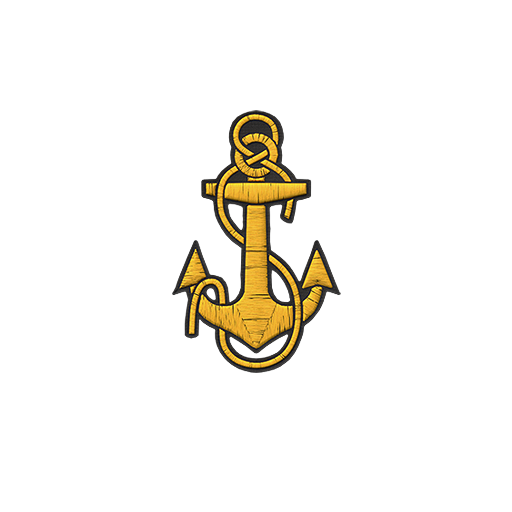# Vermento

Players

6

10758
• #### Clan

[IBERO]

• Rank
Seaman
• Insignia
[IBERO]## Recent Profile Visitors

The recent visitors block is disabled and is not being shown to other users.

1. El pequeñín de tier X
2. Bueno, ahí va el pequeñín del julio.
3.## Concurso Pugna de los Elementos - ¡¡Fuego en el Agua!

<<<<<<<<<<<<<<<<<<<<<<<<<<<<<<<<<<<<<<<<<<<<<<<<<<<<<<<<<<<<<<<<<<<<<<<<<<<<<<<<<<<<<<<<<<
4.## Concurso Pugna de los Elementos - ¡¡Fuego en el Agua! - 2ª Edición

Me apunto
5.## Me pongo de mala leche cuando juego tier X, y con razón

Completamente de acuerdo, y eso ya se empieza a ver en tier 8 y más en 9.
×

• Calendar